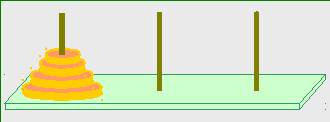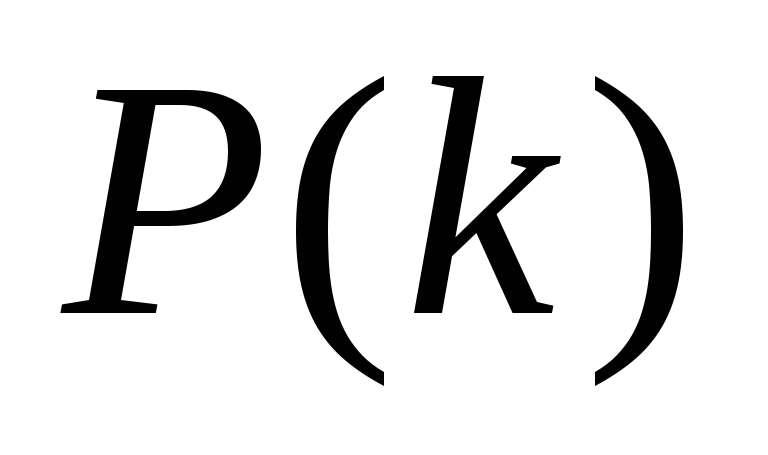# The Magnetic Tower of Hanoi

 The Magnetic Tower of Hanoi Uri Levy Atlantium Technologies, Har-Tuv Industrial Park, Israel uril@atlantium.com Abstract In this work I study a modified Tower of Hanoi puzzle, which I term Magnetic Tower of Hanoi (MToH). The original Tower of Hanoi puzzle, invented by the French mathematician Edouard Lucas in 1883, spans "base 2". That is – the number of moves of disk number k is 2^(k-1), and the total number of moves required to solve the puzzle with N disks is 2^N - 1. In the MToH puzzle, each disk has two distinct-color sides, and disks must be flipped and placed so that no sides of the same color meet. I show here that the MToH puzzle spans "base 3" - the number of moves required to solve an N+1 disk puzzle is essentially three times larger than he number of moves required to solve an N disk puzzle. The MToH comes in 3 flavors which differ in the rules for placing a disk on a free post and therefore differ in the possible evolutions of the Tower states towards a puzzle solution. I analyze here algorithms for minimizing the number of steps required to solve the MToH puzzle in its different versions. Thus, while the colorful Magnetic Tower of Hanoi puzzle is rather challenging, its inherent freedom nurtures mathematics with remarkable elegance. The Classical Tower of Hanoi The classical Tower of Hanoi (ToH) puzzle[1,2,3] consists of three posts, and N disks. The puzzle solution process ("game") calls for one-by-one disk moves restricted by one "size rule". The puzzle is solved when all disks are transferred from a "Source" Post to a "Destination" Post.Figure 1: The classical Tower of Hanoi puzzle. The puzzle consists of three posts, and N disks. The puzzle solution process ("game") calls for one-by-one disk moves restricted by one "size rule". The puzzle is solved when all disks are transferred from a "Source" Post to a "Destination" Post. The minimum number of disk-moves necessary to solve the ToH puzzle with N disks is 2N – 1. Let's define the ToH puzzle in a more rigorous way. The Classical Tower of Hanoi – puzzle description A more rigorous description of the ToH puzzle is as follows - Puzzle Components: Three equal posts A set of N different-diameter disks Puzzle-start setting: N disks arranged in a bottom-to-top descending-size order on a "Source" Post (Figure 1) Move: Lift a disk off one Post and land it on another Post Disk-placement rules: The Size Rule: A small disk can not "carry" a larger one (Never land a large disk on a smaller one) Puzzle-end state: N disks arranged in a bottom-to-top descending-size order on a "Destination" Post (one of the two originally-free posts) Given the above description of the classical ToH puzzle, let's calculate the (minimum) number of moves necessary to solve the puzzle. Number of moves Studying the classical ToH puzzle in terms of required moves to solve the puzzle, it is not too difficult to show[2,3] (prove) that the k-th disk will makemoves given by Share with your friends: